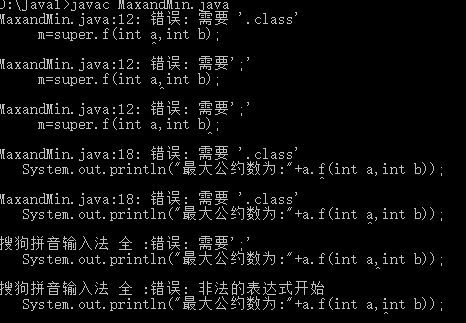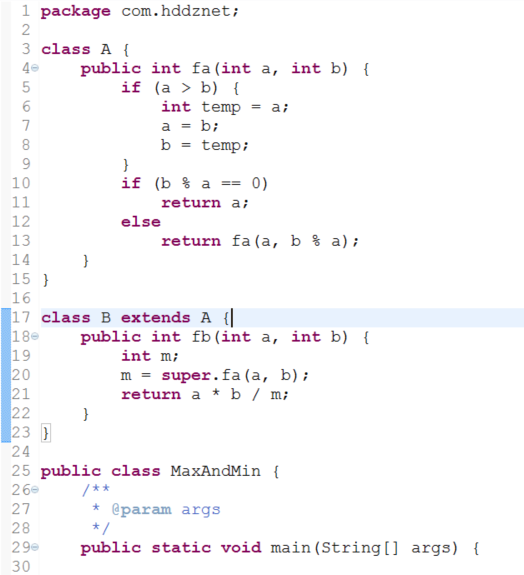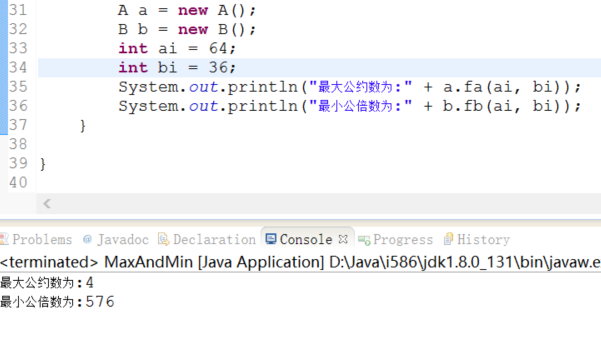java编程问题 新手求助 出现如下图问题

class A{
public int f(int a,int b){
if(a>b){
int temp=a;
a=b;
b=temp;}
if(b%a==0) return a;
else return f(a,b%a);}}
class B extends A{
public int f(int a,int b){
int m;
m=super.f(int a,int b);
return a*b/m;}}
public class MaxandMin{
public static void mian(String args[]){
A a=new A();
B b=new B();
System.out.println("最大公约数为:"+a.f(int a,int b));
System.out.println("最小公倍数为:"+b.f(int a,int b));}}5个回答

m=super.f(int a,int b);
->
m=super.f(a,b);

super和this只能放在方法调用的第一行，另外，粘代码时请使用code标签，这样代码没办法看，格式太乱了

int m;
m=super.f(a,b);
return a*b/m;}
System.out.println("最大公约数为:"+a.f(10,20));
System.out.println("最小公倍数为:"+b.f(10,20)); 这样就好了

# 存在两个问题

## 1.语法问题

1.1 方法在定义的时候，参数是带类型的；
1.2 方法在调用的时候，参数是不带类型的，需要传入的对应类型的值或者对象的应用；

## 2.覆盖父类递归方法导致的逻辑错误

2.1 子类覆盖父类的方法，默认使用子类的方法；
2.2 使用super，可调用父类被覆盖的方法；
2.3 父类递归中的方法如被子类覆盖，则调用子类的方法；fb方法（图中第20行)m=super.fa(a,b)；可不用加super，因为fa方法未被覆盖。